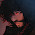## Saturday, December 1, 2012

### Numeric CAS Part 2: Binomial Expansion

Binomial Expansion

Goal: To find the coefficients of when (ax + by)^n is expanded. The variables a and b are numeric.

(ax + by)^n = Σ (n nCr k *(ax)^(n-k) * (by)^k for k = 0 to n).

Example: (3x - 2y)^3 = 27 x^3 - 54 x^2 y + 36 x y^2 - 8 y^3

For the Casio Prizm and TI-84+, each coefficient is given in order. They are also stored in List 6. For the HP 39gii, a string is built representing the expanded binomial.

The list in the above example is {27, -54, 36, -8}Casio Prizm:

POLYBINE
Binary Expansion
216 bytes

Lbl 6
"(AX+BY)^N"
"A"?→ A
"B"?→ B
"N"?→ N
If N≤0 Or Frac N≠0
Then
"N NOT AN INTEGER > 0" ◢
Goto 6
IfEnd
For 0 → K To N
ClrText
N nCr K × A ^ (N - K) × B ^ K → C
Locate 1,2,C
Locate 1,3,"×X^"
Locate 4,3,N-K
Locate 7,3,"×Y^"
Locate 10,3,K ◢
C→ List 1[K+1]
Next

Thanks to Ryan Maziarz for pointing out an extra quotation mark I had on line 3  (originally "A"?"A).  2/6/2013

Note:  The program will show the coefficients of the expansion one at a time.  You can view the entire list of the coefficients in List 1.

TI-84+:

POLYBINE
Binomial Expansion
177 bytes

: Lbl 1
: Disp "(AX+BY)^N"
: Prompt A,B,N
: If N≤0 or fPart(N)≠0
: Then
: Disp "NEED INTEGER>0"
: Pause
: Goto 1
: End
: N+1->dim(L6)
: For(K,0,N)
: N nCr K*A^(N-K)*B^K->C
: ClrHome
: Output(2,1,C)
: Output(3,1,"*X^")
: Output(3,4,N-K)
: Output(3,7,"*Y^")
: Output(3,10,K)
: Pause
: C->L6(K+1)
: End

Note:  The program will show the coefficients of the expansion one at a time.  You can view the entire list of the coefficients in L6.

HP 39gii:

POLYBINE
11/23/2012
Polynomial Binomial Expansion
Expand (ax + by)^n

The results are returned in a string. Note, if N is not a positive integer, it is converted into one.

Input: POLYBINE(A,B,C)

EXPORT POLYBINE(A,B,C)
BEGIN
LOCAL S1,S2,K;
ABS(INT(N)) → N;
"" → S1;
FOR K FROM 0 TO N DO
string(COMB(N,K)*A^(N-K)*B^K) → S2;
S1 + "+" + S2 + "*X^" + string(N-K) + "*Y^" + string(K) → S1;
END;
dim(S1) → K;
right(S1,K-1) → S1;
RETURN S1;
END;

This blog is property of Edward Shore. 2012

#### 1 comment:

1.I tried to port this to the Casio FX 9860 but it returned a syntax error. Could you kindly try to port this as maybe a .G1A file? Thanks

### Calculator Python: Lambda Functions

Calculator Python: Lambda Functions Introduction to Lambda Functions Lambda functions are a quick, one expression, one line, python function...